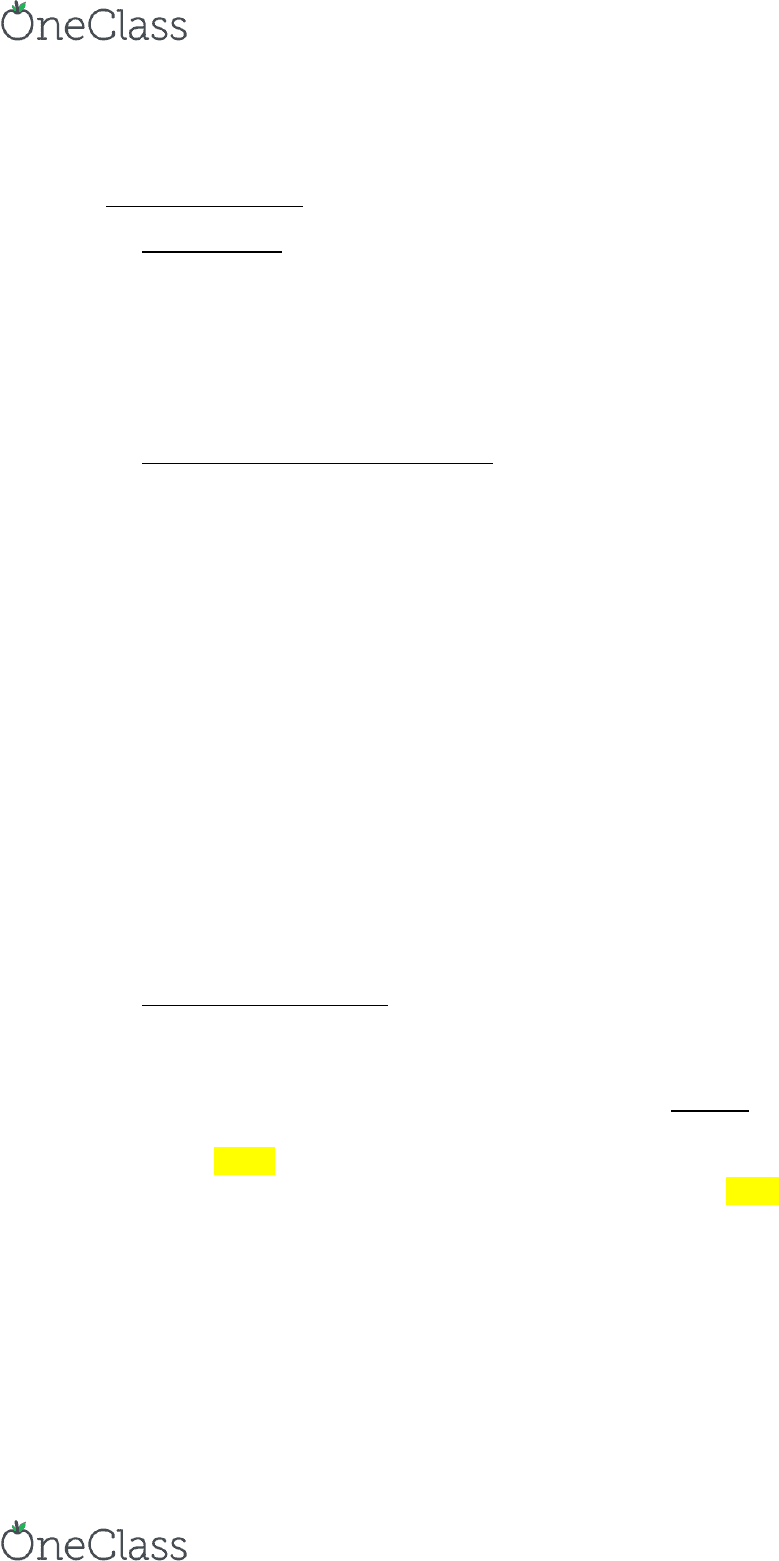# CSD 313L Lecture Notes - Lecture 2: Vocal Folds

29 views3 pagesHearing Science
1/20/2017 Day Two
What is sound?
Sound vibration of the sound source
Vocal folds vibrate to produce speech
Some vibrations are longer than others
Sound is a physical event; need a base knowledge in physics
Today’s lass ill e foused on basic physics.
Three fundamental physical quantities
1. Mass- the amount of matter that is present
Units for mass are grams(g) and kilograms(kg)
1kg = 1000g
1lb = 454g
**We’ll e usig the iteatioal syste.
Weight is related to gravity; mass is not.
Easiest eight loss egie is to go to the oo.
2. Length- the distance between two spacial points
Units for length are meter (m), kilometer (km), feet, inch, yard.
1in = 0.0254m
3. Time (t)
1 second = the time needed for light to travel 300,000km
Second is the standard unit for time (s). Also millisecond (ms).
1s = 1000ms
Ay othe physial uatity ill oe fo these thee. Aythig else is alled a deied
physial uatity.
Derived physical quantities
4. Displacement (d) the change in the special position ( A B or A B )
Even though the distance between A and B may be the same as the distance between B and
A, they have different directions and are therefore different.
Displaeet is a uatity that is dietio sesitie.
Vector a physical quantity that needs both _______ and direction.
**A uatity that does’t ae aout dietio is alled a scalar.
Displacement is a vector.
Unit is m, km, or cm.
5. Velocity (v) the displacement in 1 second.
**V = d/t (answer will be in m/s)
1mph = 1609 m 1hr = 60 x 60 = 3600s 1 mile/hour = 1609/3600 0.45m/s 66.7 x
0.45 m/s 30.015 m/s
Velocity is a vector
find more resources at oneclass.com
find more resources at oneclass.com
Unlock document

This preview shows page 1 of the document.
Unlock all 3 pages and 3 million more documents.

## Document Summary

Sound vibration of the sound source. Sound is a physical event; need a base knowledge in physics. Today"s (cid:272)lass (cid:449)ill (cid:271)e fo(cid:272)used on basic physics. Three fundamental physical quantities (cid:862)easiest (cid:449)eight loss (cid:396)egi(cid:373)e is to go to the (cid:373)oo(cid:374). (cid:863) Mass- the amount of matter that is present. Units for mass are grams(g) and kilograms(kg) Weight is related to gravity; mass is not. Units for length are meter (m), kilometer (km), feet, inch, yard. 1 second = the time needed for light to travel 300,000km. Second is the standard unit for time (s). A(cid:374)y othe(cid:396) physi(cid:272)al (cid:395)ua(cid:374)tity (cid:449)ill (cid:272)o(cid:373)e f(cid:396)o(cid:373) these th(cid:396)ee. A(cid:374)ythi(cid:374)g else is (cid:272)alled a (cid:862)de(cid:396)i(cid:448)ed physi(cid:272)al (cid:395)ua(cid:374)tity. (cid:863) Displacement (d) the change in the special position ( a b or a b ) Even though the distance between a and b may be the same as the distance between b and.

## Get access

\$10 USD/m
Billed \$120 USD annuallyHomework Help
Study Guides
Textbook Solutions
Class Notes
Textbook Notes
Booster Class